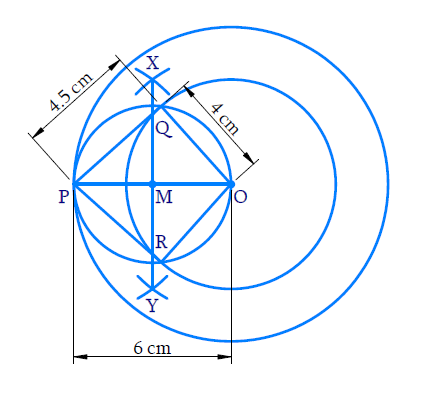# Ex.11.2 Q2 Constructions Solution - NCERT Maths Class 10

Go back to  'Ex.11.2'

## Question

Construct a tangent to a circle of radius $$4 \,\rm{cm}$$ from a point on the concentric circle of radius $$6 \,\rm{cm}$$ and measure its length. Also verify the measurement by actual calculation.

Video Solution
Constructions
Ex 11.2 | Question 2

## Text Solution

#### Steps:Steps of construction:

(i) Take $$‘O’$$ as centre and radius $$4 \,\rm{cm}$$ and $$6\,\rm{cm}$$ draw two circles.

(ii) Take a point $$‘P’$$ on the bigger circle and join $$OP$$.

(iii) With $$‘O’$$ and $$‘P’$$ as centre and radius more than half of $$OP$$ draw arcs above and below $$OP$$ to intersect at $$X$$ and $$Y$$.

(iv) Join $$XY$$ to intersect $$OP$$ at $$M$$.

(v) With $$M$$ as centre and $$OM$$ as radius draw a circle to cut the smaller circle at $$Q$$ and $$R.$$

(vi) Join $$PQ$$ and $$PR$$.

$$PQ$$ and $$PR$$ are the required tangent where \begin{align}\rm{PQ}=4.5\end{align} (aprox)

Proof:

$$\angle {\rm{PQO}} = {90^ \circ }$$ (Angle in a semi - circle)

$$\therefore$$\begin{align} P Q \perp O Q\end{align}

$$OQ$$ being the radius of the smaller circle, $$PQ$$ is the tangent at $$Q$$.

In right $${\rm{\Delta PQO,}}$$

$$OP = 6\,\rm{cm}$$ (radius of the bigger circle)

$$OQ = 4 \,\rm{cm}$$ (radius of the smaller circle)

\begin{align} {PQ}^{2} &=({OP})^{2}-(\mathrm{OQ})^{2} \\ &=(6)^{2}-(4)^{2} \\ &=36-16 \\ &=20 \end{align}

\begin{align} {{PQ }}&=\sqrt {{\rm{20}}} \\ &={{4.5}}\,\,\,{\rm{(approx)}} \end{align}
Similarly, $${\rm{PR = 4}}{\rm{.5 }}\left( {{\rm{approx}}{\rm{.}}} \right)$$

Learn from the best math teachers and top your exams

• Live one on one classroom and doubt clearing
• Practice worksheets in and after class for conceptual clarity
• Personalized curriculum to keep up with school